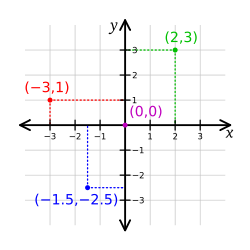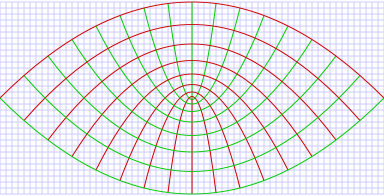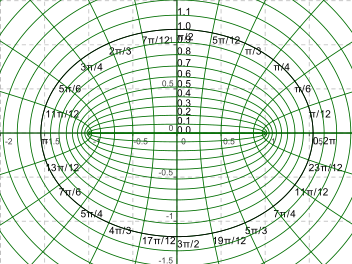Two Dimensional Coordinate Systems are a method of describing a point in a plane using an ordered pair of numbers $(n_1,n_2)$ called coordinates such that $n_1$ = constant is a set of non-intersecting lines (or curves). Similarly, $n_2$ = constant produce a second set of non-intersecting lines (or curves). Therefore the ordered pair of numbers indicate a point located at the intersection of a pair of the lines (or curves), one from each set.

A special class of coordinate systems are those where each line of one set intersect at right angles to every line of the other set. Such a system is called an Orthogonal Coordinate System.

Here are some orthogonal coordinate systems in two dimensionsCartesian Coordinate System - Coordinate curves are both straight lines - named after the inventor of the system René DescartesPolar Coordinate System - Coordinate curves are circles and straight linesParabolic Coordinate System - Coordinate curves are both parabolasBipolar Coordinate System - Coordinate curves are both circlesElliptic Coordinate System - Coordinate curves are ellipses and hyperbolae

By extension Coordinate Systems exist for three and more dimensions.